Mathematical Calculators

# Trigonometry Calculator

Calculate easily trigonometric values Sin, Cos, Tan, Cot, Sec and Csc with our free online calculator!

#### Trigonometry calculator

 ◦What is trigonometry? ◦What are the primary or basic trigonometric functions? ◦What are reciprocal trigonometric functions? ◦What is trigonometry used for? ◦Real-life applications of trigonometry

## What is trigonometry?

Trigonometry is a branch of mathematics dealing with the sides and the angles in a triangle. This subject also covers the relevant functions used for the calculation of angles.
Trigonometry - Wikipedia

## What are the primary or basic trigonometric functions?

Below you will see a list of the primary trigonometric functions that are mainly used when utilising trigonometry.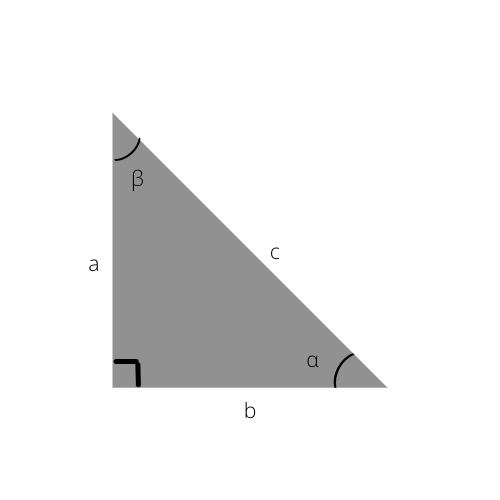An example of a right triangle. The "α" angle in this image is referenced in the following formulas.

### 1) Sine of an angle

Formula: sin α = opposite / hypotenuse
The ratio of the sine of an angle, in the context of a right triangle, is the length of the opposite divided by the length of the hypotenuse.

### 2) Cosine of an angle

Formula: cos α = adjacent / hypotenuse
The cosine of an angle, in the context of a right triangle, is calculated by dividing the length of the adjacent by the length of the hypotenuse.

### 3) Tangent of an angle

Formula: tan α = sin α / cos α = opposite / adjacent
The tangent of an angle, in the context of a right triangle, is calculated by dividing the sine by the cosine. This can also be substituted by dividing the opposite by the adjacent.
Also, keep in mind the trigonometric table when utilising these functions.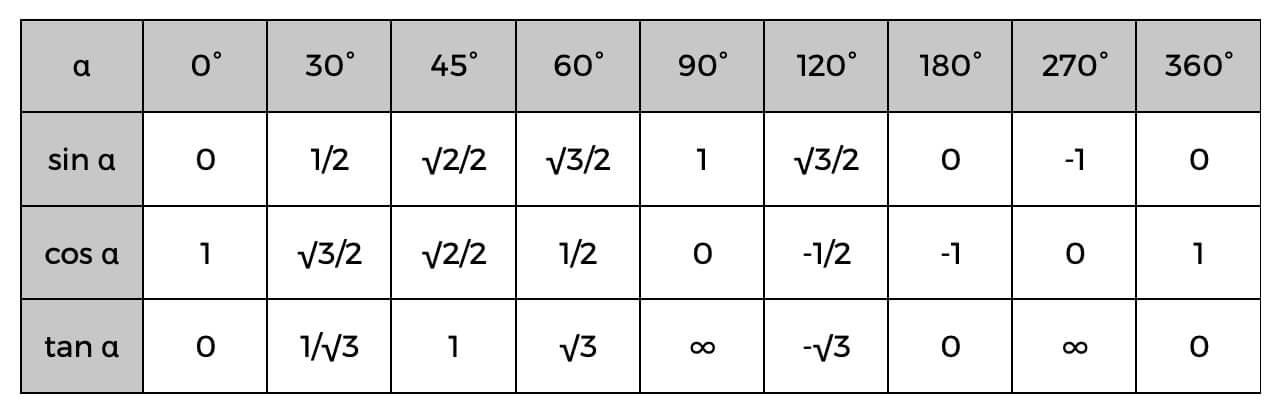The trigonometric table

## What are reciprocal trigonometric functions?

Besides the primary trigonometric functions, there is also another set of functions that are not as used compared to the first category. These include secant (sec), cosecant (csc), and cotangent (cot).
secant - sec α = 1 / cos α
cosecant - csc α = 1 / sin α
cotangent - cot α = 1 / tan α = cos α / sin α

## What is trigonometry used for?

Trigonometry is related to triangles, in particular right triangles. So, wherever you see a triangle outside of the mathematics world, you bet that trigonometry is helpful. An example of this is the use of trigonometric calculations in fields such as architecture, astronomy, and chemical engineering.

## Real-life applications of trigonometry

Other than the obvious use of trigonometry, which is in mathematics, trigonometry is also used in real-life situations and fields.

### 1) Architecture and engineering

It is not too far off to think about the usage of trigonometric functions in architecture. These functions are mostly used to calculate the length of the diagonal connection that connects two lines. This is used to calculate the diagonal length of a roof's slope when designing a sloped roof. You would only need to know the height and length of the roof and you're good to go!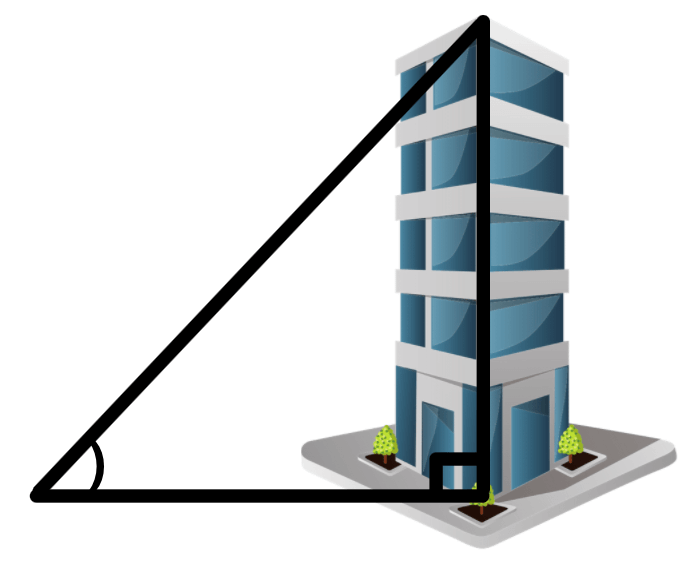### 2) Astronomy

Astronomy is an important subject to which old cultures mostly paid attention. When speaking of this, probably one of the first things that come to mind is the constellations and calculating their distance from each other and other objects in space, which is one of the more simple uses of astronomy. In other words, trigonometry can be used to calculate the distance of the sun and the earth from different stars. The distance of the stars is important for calculating their factors in the world of astronomy.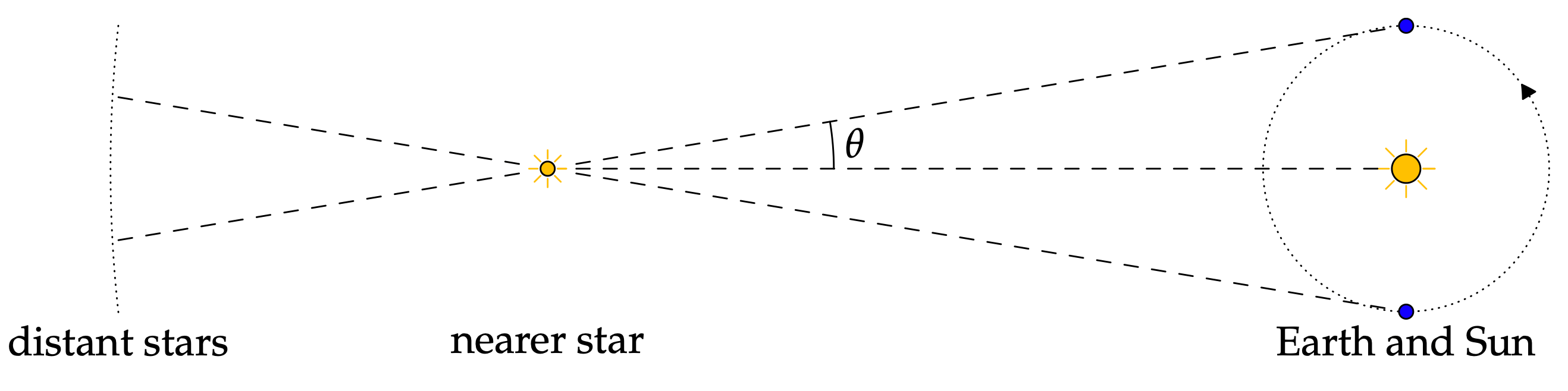See this article for further information on astronomy and its relation to trigonometry:
Astrologys relation to trigonometry

### 3) Electronics and electrical engineering

Trigonometry is used for resolving math problems in electronics and electrical engineering, mostly when designing a model. Another example of the importance is when making aesthetic additions and making sure they don't disturb the function of the model.
However, trigonometric functions come in very handy when working with circuits. See the below visual example for further demonstration and to get an idea of how trigonometry translates into circuit logic.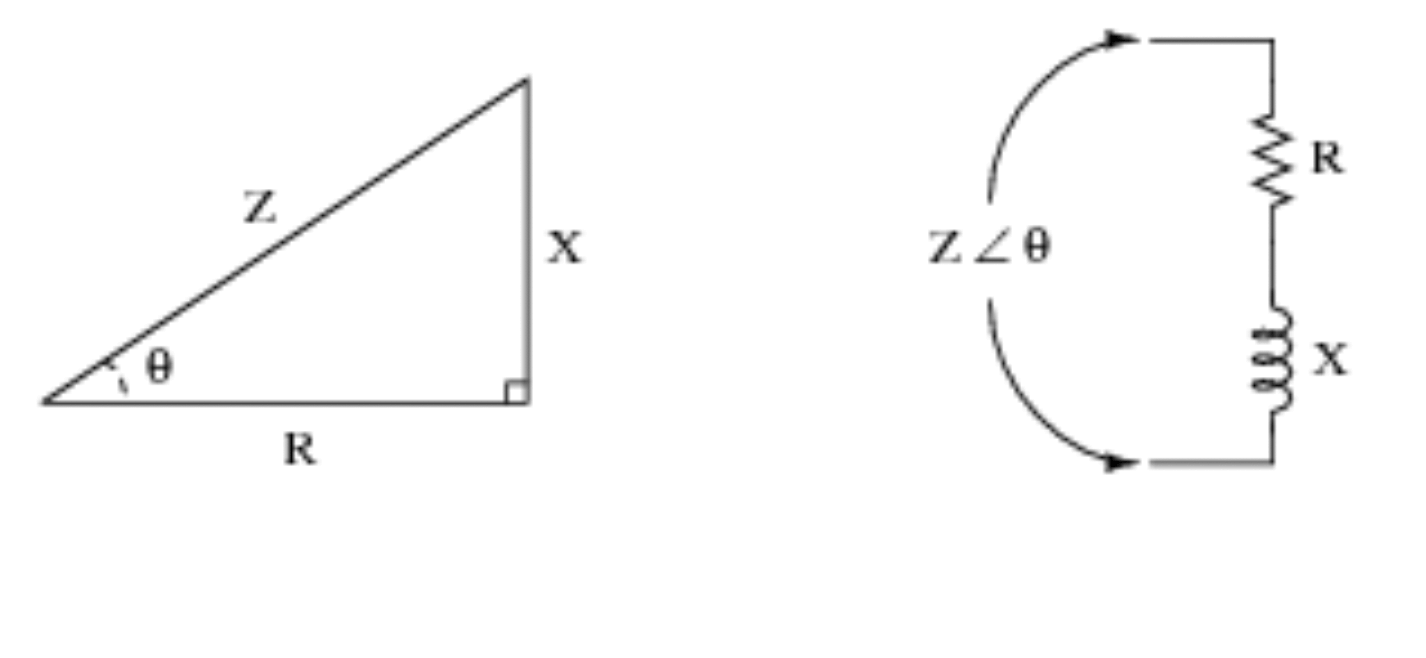### 4) Seismology

Seismology is the study of earthquakes, as well as the seismic waves that move through and around the earth. Trigonometry comes in to ease the calculation of the vertical and horizontal distance a seismic wave travels.### 5) Land surveying (civil engineering)

Surveying has been a profession that has been around for a long time, at least as long as recorded history shows. This is done by a surveyor who has the job of accurately measuring the earth's surfaces on a large scale. You might have guessed the use of trigonometry by now; basically, trigonometry comes in when the surveyor needs to calculate the length, areas, and relative angles between the objects on the landscape.
The example below is a good visual demonstrator of what has been previously explained. A surveyor uses trigonometric fractions to calculate his/her own distance from the mountain top or from anywhere else they pick.Surveying - WikipediaArticle author
Parmis Kazemi
Parmis is a content creator who has a passion for writing and creating new things. She is also highly interested in tech and enjoys learning new things.

###### Trigonometry Calculator English
Published: Fri Oct 29 2021
In category Mathematical calculators